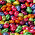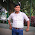# Get STM32 tutorial using HAL at \$10 for a limited time!

## Friday, January 1, 2016

### ARM Cortex-M3 (STM32F103) Tutorial - GPIO - Control LED On/Off

In this tutorial, I will explain how to use STM32F103 GPIO peripheral for controlling a LED. GPIO (General Purpose Input Output) on STM32F103 can be configure to 4 different modes (input mode, output mode, analog input mode, alternate function mode). For controlling LED on/off from GPIO, we need to configure GPIO in output mode.

There are 2 output modes on STM32F103. Their modes are output open drain and output push-pull. The logic voltage of STM32F103 is 3.3V, so their port bit output voltage is 3.3V. This is the characteristic of I/O port bit when configured as output mode:

• The output buffer is enabled:
• Open drain mode: a "0" in the output register activates the N-MOS while a "1" in the output register leaves the port in Hi-Z. (the P-MOS is never activated).
• Push-pull mode: a "0" in the output register activates the N-MOS while a "1" in the output register activates the P-MOS.
• The schmitt trigger input is activated.
• The weak pull-up and pull-down resistors are disabled.
• The data present on the I/O pin is sampled into the input data register every APB2 clock cycle.
• A read access to the input data register gets the I/O state in open drain mode.
• A read access to the output data register gets the last written value in push-pull mode.

The LED circuit that I use in this tutorial is like this:

The LED configuration is active low, so when the PA0 is low or "0" the LED will turn on. When the PA0 is high or "1" the LED will turn off. The output mode that I use is output push-pull. So when I write "0" to output register, the N-MOS will turn on, therefore the LED will turn on (current can flow from Vdd to Vss through LED and resistor). When I write "1" to output register, the P-MOS will turn on, therefore the LED will turn off (current can't flow from Vdd to Vdd).

To configure PA0 as an output push-pull, we must do this steps:
1. You must enable the peripheral clock for GPIOA. GPIOA is connected to APB2 bus.
2. Initialize GPIOA by using GPIO_InitTypeDef struct data type.
This the code to make a LED on/off within a period of time (LED blinking):
```#include "stm32f10x.h"
#include "stm32f10x_rcc.h"
#include "stm32f10x_gpio.h"

void delay(unsigned int nCount);
GPIO_InitTypeDef GPIO_InitStruct;

int main (void)
{
// Enable clock for GPIOA
RCC_APB2PeriphClockCmd(RCC_APB2Periph_GPIOA, ENABLE);

// Configure PA0 as push-pull output
GPIO_InitStruct.GPIO_Pin = GPIO_Pin_0;
GPIO_InitStruct.GPIO_Speed = GPIO_Speed_2MHz;
GPIO_InitStruct.GPIO_Mode = GPIO_Mode_Out_PP;
GPIO_Init(GPIOA, &GPIO_InitStruct);

while (1)
{
/* Toggle LED on PA0 */
// Reset bit will turn on LED (because the logic is interved)
GPIO_ResetBits(GPIOA, GPIO_Pin_0);
delay(1000);
// Set bit will turn off LED (because the logic is interved)
GPIO_SetBits(GPIOA, GPIO_Pin_0);
delay(1000);
}
}

// Delay function
void delay(unsigned int nCount)
{
unsigned int i, j;

for (i = 0; i < nCount; i++)
for (j = 0; j < 0x2AFF; j++);
}
```
It is also possible to use output open drain mode to control the LED. The LED circuit is the same. So when I write "0" to output register, the N-MOS will turn on, therefore the LED will turn on (current can flow from Vdd to Vss through LED and resistor). When I write "1" to output register PA0 is in Hi-Z (current can't flow because PA0 is Hi-Z/floating), therefore the LED will turn off.

1.2.